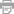Here We Are Now Letra

Hannah Grace

Remedy

Letra de Here We Are Now
I don’t wanna live in the mirror
Tired of waiting, for my reflection to change

Tryna find a way to see clearer
And leave all my worries behind

Here we are now, here we are now, here we are
Here we are now, here we are now, here we are

So we breath, and we dance, and we let go
Till we’re free, in the light, in the everglow
Here we are now, here we are now, here we are
Here we are now, here we are now, here we are

I just wanna live in the moment
Under the surface I promised myself I’d be brave
For the first time I need no one
To wash all my troubles away

Here we are now, here we are now, here we are
Here we are now, here we are now, here we are

So we breath, and we dance, and we let go
Till we’re free, in the light, in the everglow
Here we are now, here we are now, here we are
Here we are now, here we are now, here we are
Maybe we just talk too much
But never really say enough
Maybe we just talk too much
But never really say enough
Maybe we just talk too much
But never really say enough
Maybe we just talk too much
But never really say enough

So we breath, and we dance, and we let go
Till we’re free, in the light, in the everglow
Here we are now, here we are now, here we are
Here we are now, here we are now, here we are
•Imprimir Letra